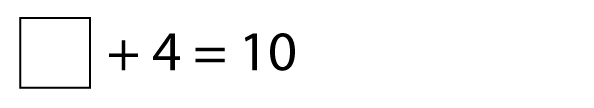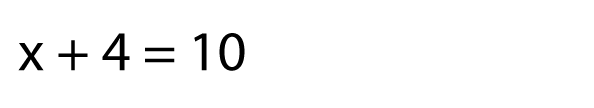What is algebra?

Algebra is like solving a puzzle. Here’s an example of a puzzle we can solve.In algebra, we don't use empty boxes like this. Instead, we use letters. Usually, we use the letters x and y. Letters take up less space than empty boxes!

So this puzzle would become:The letter x represents the empty box, it's the missing number.

Question

x + 4 = 10

x = ?

Solution

? + 4 = 10

6 + 4 = 10

The missing number is 6.

Answer: x = 6